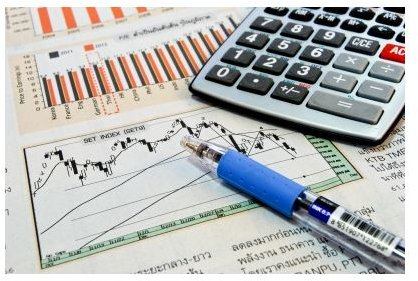# What Is the Difference Between Amortization and Depreciation?Any asset that is a physical object is a tangible asset. Tangible assets are depreciated on the company's financial statements. Intangible assets may include such things as goodwill, trademarks, copyrights, technology, franchises, licenses, and patents. Sometimes, they are derived from the internal work of the company do not have an acquisition cost. Intangible assets are an important resource for companies and are often evidenced by a legal document. These assets are amortized instead of depreciated. So when what's the difference between amortization and depreciation when it comes to assets?

## Calculating Amortization Expense

If an intangible asset does have a historical acquisition cost from purchase, this cost is used in amortization. If these assets are developed within the company, costs incurred during development may be expensed as they are incurred. If the life of an intangible asset can be determined, then the straight-line method is a common method of amortization expense.

Straight-Line

A patent purchased for \$100,000 and expected to be used for 20 years, would be expensed at \$5,000 per year (\$100,000/20).

If the useful life of an intangible asset can not be determined, then these assets are not necessarily amortized. It is assumed that these intangibles will carry their value indefinitely, unless impairment occurs. Impairment is an accounting term used when the book value of an intangible assets exceeds possible future cash flows. If the book value is more than is expected to be received from the intangible asset, then an expense is calculated as the current book value, less the current market value of the asset. This expense is recorded on the books as loss due to impairment.

## Calculating Depreciation Expense

Unlike amortization, depreciation is calculated on tangible company assets. Examples of tangible assets are plant equipment, vehicles, and buildings. Common methods of depreciation include the straight-line, double declining balance, and units of production methods. When calculating depreciation, care should be taken to match the expected revenues from the asset closely to the depreciation expense. An asset expected to generate higher income in the early years of its life would be best suited using the accelerated method of depreciation. Its depreciation expense would be higher in the years that income generated from the asset was higher. Assets expected to bring in consistent revenues throughout their useful life should be expensed through the straight-line depreciation method. The units of production method of depreciation is often used when a total output produced by an asset over its useful life is easily determined. The formula used for this method of depreciation ties the depreciation expense to the assets units of output.

Simply put, the difference between amortization and depreciation is the tangibility of the asset. Assets that aren't tangible may be amortized, but only if their book value is expected to decline over time, or expenses are incurred on behalf of the asset. Depreciation is calculated for all tangible assets that decline in value over time. Land is the only tangible asset that isn't commonly expected to depreciate in value.